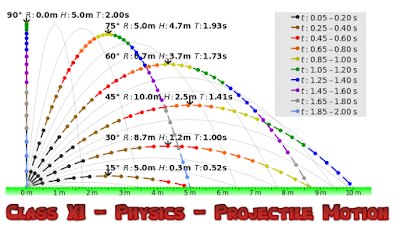## Class XI - PHYSICS - PROJECTILE MOTION - (Assignment)image credits: cmglee (Wikimedia)

1. An aeroplane is flying in horizontal direction with a velocity of 600 km/h and at a height of 1960 m. When it is vertically above the point A on the ground, a body is dropped from it. The body strikes the ground at a point B. Calculate distance AB.

2. A body is thrown horizontally from the top of a lower and strike the ground in 3 seconds at an angle 45° with the horizontal. Find the height of the lower and the speed with which it was projected.
[Answer:  44.1 m , 29.4 m/s ]

3. An air craft is flying at a height of 3,500 m above the ground. If the angle subtended at a ground observation point by the air craft positions 10 s apart is 30° while passing over his head, what is the speed of the air craft?
[Answer: 187.5 m/s ]

4. A ball is thrown horizontally from the top of a lower with a speed of 50 m/s. Find the velocity and position at the end of 3 second g = 9.8 ms⁻².
[Answer: 58 m/s 30° 27' with horizontal ]

5. A ball is projected from ground with a velocity of 19.6 m/s at an angle of 30 0 with the horizontal. Determine its time of flight and the horizontal distance it travels.
[Answer:  2s , 33.95m ]

6. Prove that the maximum horizontal range is 4 times the maximum height attained by a projectile which is fired along the required oblique direction.

7. Find the minimum velocity with which the horizontal range is 39.2 m.
[Answer: 19.6 m/s ]

8. The ceiling of a long hall is 25 m high. What is the maximum horizontal distance that the ball thrown with a speed of 40 m/s can go without hitting the ceiling of the hall.
[Answer: 150.5 m ]

9. Show that the maximum range of a projectile in any direction is described in the same time in which it would fall freely under gravity through this distance starting from rest.

10. A target is fixed the top of a pole 13 m high. A person standing at distance 50 m from the pole is capable of projecting a stone with a velocity 10√g m/s. If he wants to strike the target in shortest possible time at what angle should be project the stone.

11. A projectile is projected at time t = 0 , from a point P on the ground with a speed v₀ at an angle 45° to the horizontal. Find the angular momentum of the particle about the point P at time t = v₀/g.
[Answer: mv₀³ /{2√2g} ]

12. A grass hopper can jump a maximum horizontal distance of 20.4 cm. If it spends negligible time on the ground, what is its speed of travel along the road (Take g = 10 m/s²).
[Answer:  1 m/s ]

13. A body is projected such that its kinetic energy at the top is 3/4th of its initial kinetic energy. What is the initial angle of projection of the projectile with the horizontal?

14. A hunter aims his gun and fires a bullet leaves the barrel of the gun, the monkey dropped. Will the bullet hit the monkey? Substantiate your answer with proper reasoning.

15. A cricketer can throw a ball to a maximum horizontal distance of 100 m. With the same speed how high above the ground can the cricket throw the same ball.
[Answer: 50 m ]

16. Calculate the angular velocity of the minute’s hand of a clock.

17. A body is moving in a circle of radius 100 cm with a time period of 2 second. Find the acceleration.